mscroggs.co.uk
mscroggs.co.uksubscribe

# Blog

2019-04-09
In the latest issue of Chalkdust, I wrote an article with Edmund Harriss about the Harriss spiral that appears on the cover of the magazine. To draw a Harriss spiral, start with a rectangle whose side lengths are in the plastic ratio; that is the ratio $$1:\rho$$ where $$\rho$$ is the real solution of the equation $$x^3=x+1$$, approximately 1.3247179.
This rectangle can be split into a square and two rectangles similar to the original rectangle. These smaller rectangles can then be split up in the same manner.
Drawing two curves in each square gives the Harriss spiral.
This spiral was inspired by the golden spiral, which is drawn in a rectangle whose side lengths are in the golden ratio of $$1:\phi$$, where $$\phi$$ is the positive solution of the equation $$x^2=x+1$$ (approximately 1.6180339). This rectangle can be split into a square and one similar rectangle. Drawing one arc in each square gives a golden spiral.
The golden and Harriss spirals are both drawn in rectangles that can be split into a square and one or two similar rectangles.
Continuing the pattern of these arrangements suggests the following rectangle, split into a square and three similar rectangles:
Let the side of the square be 1 unit, and let each rectangle have sides in the ratio $$1:x$$. We can then calculate that the lengths of the sides of each rectangle are as shown in the following diagram.
The side lengths of the large rectangle are $$\frac{1}{x^3}+\frac{1}{x^2}+\frac2x+1$$ and $$\frac1{x^2}+\frac1x+1$$. We want these to also be in the ratio $$1:x$$. Therefore the following equation must hold:
$$\frac{1}{x^3}+\frac{1}{x^2}+\frac2x+1=x\left(\frac1{x^2}+\frac1x+1\right)$$
Rearranging this gives:
$$x^4-x^2-x-1=0$$ $$(x+1)(x^3-x^2-1)=0$$
This has one positive real solution:
$$x=\frac13\left( 1 +\sqrt{\tfrac12(29-3\sqrt{93})} +\sqrt{\tfrac12(29+3\sqrt{93})} \right).$$
This is equal to 1.4655712... Drawing three arcs in each square allows us to make a spiral from a rectangle with sides in this ratio: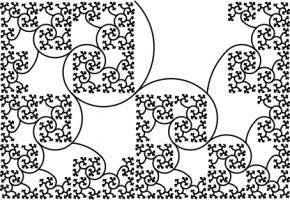A spiral which may or may not have a name yet.

### Continuing the pattern

The side lengths of the largest rectangle are $$1+\frac2x+\frac3{x^2}+\frac1{x^3}+\frac1{x^4}$$ and $$1+\frac2x+\frac1{x^2}+\frac1{x^3}$$. Looking for the largest rectangle to also be in the ratio $$1:x$$ leads to the equation:
$$1+\frac2x+\frac3{x^2}+\frac1{x^3}+\frac1{x^4} = x\left(1+\frac2x+\frac1{x^2}+\frac1{x^3}\right)$$ $$x^5+x^4-x^3-2x^2-x-1 = 0$$
This has one real solution, 1.3910491... Although for this rectangle, it's not obvious which arcs to draw to make a spiral (or maybe not possible to do it at all). But at least you get a pretty fractal: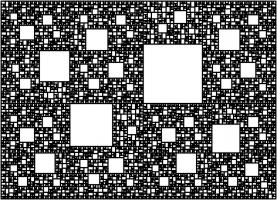### Continuing the pattern

We could, of course, continue the pattern by repeatedly adding more rectangles. If we do this, we get the following polynomials and solutions:
 Number of rectangles Polynomial Solution 1 $$x^2 - x - 1=0$$ 1.618033988749895 2 $$x^3 - x - 1=0$$ 1.324717957244746 3 $$x^4 - x^2 - x - 1=0$$ 1.465571231876768 4 $$x^5 + x^4 - x^3 - 2x^2 - x - 1=0$$ 1.391049107172349 5 $$x^6 + x^5 - 2x^3 - 3x^2 - x - 1=0$$ 1.426608021669601 6 $$x^7 + 2x^6 - 2x^4 - 3x^3 - 4x^2 - x - 1=0$$ 1.4082770325090774 7 $$x^8 + 2x^7 + 2x^6 - 2x^5 - 5x^4 - 4x^3 - 5x^2 - x - 1=0$$ 1.4172584399350432 8 $$x^9 + 3x^8 + 2x^7 - 5x^5 - 9x^4 - 5x^3 - 6x^2 - x - 1=0$$ 1.412713760332943 9 $$x^{10} + 3x^9 + 5x^8 - 5x^6 - 9x^5 - 14x^4 - 6x^3 - 7x^2 - x - 1=0$$ 1.414969877544769
The numbers in this table appear to be heading towards around 1.414, or $$\sqrt2$$. This shouldn't come as too much of a surprise because $$1:\sqrt2$$ is the ratio of the sides of A$$n$$ paper (for $$n=0,1,2,...$$). A0 paper can be split up like this:
This is a way of splitting up a $$1:\sqrt{2}$$ rectangle into an infinite number of similar rectangles, arranged following the pattern, so it makes sense that the ratios converge to this.

### Other patterns

In this post, we've only looked at splitting up rectangles into squares and similar rectangles following a particular pattern. Thinking about other arrangements leads to the following question:
Given two real numbers $$a$$ and $$b$$, when is it possible to split an $$a:b$$ rectangle into squares and $$a:b$$ rectangles?
If I get anywhere with this question, I'll post it here. Feel free to post your ideas in the comments below.

### Similar posts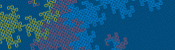Dragon curves IITMiP 2019 treasure punt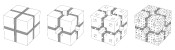Christmas card 2018World Cup stickers 2018, pt. 3

Comments in green were written by me. Comments in blue were not written by me.
2019-05-02
@g0mrb: CORRECTION: There seems to be no way to correct the glaring error in that comment. A senior moment enabled me to reverse the nomenclature for paper sizes. Please read the suffixes as (n+1), (n+2), etc.
2019-05-02
I shall remain happy in the knowledge that you have shown graphically how an A(n) sheet, which is 2 x A(n-1) rectangles, is also equal to the infinite series : A(n-1) + A(n-2) + A(n-3) + A(n-4) + ... Thank-you, and best wishes for your search for the answer to your question.

Allowed HTML tags: <br> <a> <small> <b> <i> <s> <sup> <sub> <u> <spoiler> <ul> <ol> <li>
To prove you are not a spam bot, please type "e" then "q" then "u" then "a" then "t" then "i" then "o" then "n" in the box below (case sensitive):
2018-07-07
So you've calculated how much you should expect the World Cup sticker book to cost and recorded how much it actually cost. You might be wondering what else you can do with your sticker book. If so, look no further: this post contains 5 mathematical things involvolving your sticker book and stickers.Stickers 354 and 369: Alisson and Roberto Firmino
In a group of 23 people, there is a more than 50% chance that two of them will share a birthday. This is often called the birthday paradox, as the number 23 is surprisingly small.
Back in 2014 when Alex Bellos suggested testing the birthday paradox on World Cup squads, as there are 23 players in a World Cup squad. I recently discovered that even further back in 2012, James Grime made a video about the birthday paradox in football games, using the players on both teams plus the referee to make 23 people.
In this year's sticker book, each player's date of birth is given above their name, so you can use your sticker book to test it out yourself.

One of the cities in which games are taking place in this World Cup is Kaliningrad. Before 1945, Kaliningrad was called Königsberg. In Königsburg, there were seven bridges connecting four islands. The arrangement of these bridges is shown below.
The people of Königsburg would try to walk around the city in a route that crossed each bridge exactly one. If you've not tried this puzzle before, try to find such a route now before reading on...
In 1736, mathematician Leonhard Euler proved that it is in fact impossible to find such a route. He realised that for such a route to exist, you need to be able to pair up the bridges on each island so that you can enter the island on one of each pair and leave on the other. The islands in Königsburg all have an odd number of bridges, so there cannot be a route crossing each bridge only once.
In Kaliningrad, however, there are eight bridges: two of the original bridges were destroyed during World War II, and three more have been built. Because of this, it's now possible to walk around the city crossing each bridge exactly once.
A route around Kaliningrad crossing each bridge exactly once.
I wrote more about this puzzle, and using similar ideas to find the shortest possible route to complete a level of Pac-Man, in this blog post.

### Sorting algorithms

If you didn't convince many of your friends to join you in collecting stickers, you'll have lots of swaps. You can use these to practice performing your favourite sorting algorithms.

#### Bubble sort

In the bubble sort, you work from left to right comparing pairs of stickers. If the stickers are in the wrong order, you swap them. After a few passes along the line of stickers, they will be in order.
Bubble sort

#### Insertion sort

In the insertion sort, you take the next sticker in the line and insert it into its correct position in the list.
In the quick sort, you pick the middle sticker of the group and put the other stickers on the correct side of it. You then repeat the process with the smaller groups of stickers you have just formed.

### The football

Sticker 007 shows the official tournament ball. If you look closely (click to enlarge), you can see that the ball is made of a mixture of pentagons and hexagons. The ball is not made of only hexagons, as road signs in the UK show.
Stand up mathematician Matt Parker started a petition to get the symbol on the signs changed, but the idea was rejected.
If you have a swap of sticker 007, why not stick it to a letter to your MP about the incorrect signs as an example of what an actual football looks like.

### Psychic pets

Speaking of Matt Parker, during this World Cup, he's looking for psychic pets that are able to predict World Cup results. Why not use your swaps to label two pieces of food that your pet can choose between to predict the results of the remaining matches?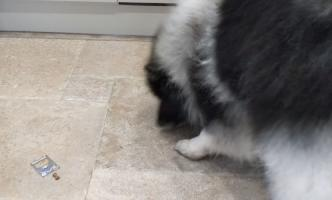Timber using my swaps to wrongly predict the first match

### Similar postsWorld Cup stickers 2018, pt. 2World Cup stickers 2018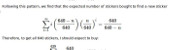World Cup stickersEuro 2016 stickers

Comments in green were written by me. Comments in blue were not written by me.
2019-06-19
@Matthew: Thank you for the calculations. Good job I ordered the stickers I wanted #IRN. 2453 stickers - that's more than the number you bought (1781) to collect all stickers!
2019-05-29
@Milad: Here is how I calculated it:

You want a specific set of 20 stickers. Imagine you have already $$n$$ of these. The probability that the next sticker you buy is one that you want is
$$\frac{20-n}{682}.$$
The probability that the second sticker you buy is the next new sticker is
$$\mathbb{P}(\text{next sticker is not wanted})\times\mathbb{P}(\text{sticker after next is wanted})$$
$$=\frac{662+n}{682}\times\frac{20-n}{682}.$$
Following the same method, we can see that the probability that the $$i$$th sticker you buy is the next wanted sticker is
$$\left(\frac{662+n}{682}\right)^{i-1}\times\frac{20-n}{682}.$$
Using this, we can calculate the expected number of stickers you will need to buy until you find the next wanted one:
$$\sum_{i=1}^{\infty}i \left(\frac{20-n}{682}\right) \left(\frac{662+n}{682}\right)^{i-1} = \frac{682}{20-n}$$
Therefore, to get all 682 stickers, you should expect to buy
$$\sum_{n=0}^{19}\frac{682}{20-n} = 2453 \text{ stickers}.$$
2019-05-16
@Matthew: Yes, I would like to know how you work it out please. I believe I have left my email address in my comment. It seems like a lot of stickers if you are just interested in one team.
2019-03-08
@Milad: Following a similiar method to this blog post, I reckon you'd expect to buy 2453 stickers (491 packs) to get a fixed set of 20 stickers. Drop me an email if you want me to explain how I worked this out.
2019-03-07
Thanks for the maths, but I have one probability question. How many packs would I have to buy, on average, to obtain a fixed set of 20 stickers?

Allowed HTML tags: <br> <a> <small> <b> <i> <s> <sup> <sub> <u> <spoiler> <ul> <ol> <li>
To prove you are not a spam bot, please type "graph" in the box below (case sensitive):
2018-07-06
This is a post I wrote for round 1 of The Aperiodical's Big Internet Math-Off 2018, where Mathsteroids lost to MENACE.
A map projection is a way of representing the surface of a sphere, such as the Earth, on a flat surface. There is no way to represent all the features of a sphere on a flat surface, so if you want a map that shows a certain feature of the world, then you map will have to lose some other feature of the world in return.
To show you what different map projections do to a sphere, I have created a version of the game Asteroids on a sphere. I call it Mathsteroids. You can play it here, or follow the links below to play on specific projections.

### Mercator projection

The most commonly used map projection is the Mercator projection, invented by Gerardus Mercator in 1569. The Mercator projection preserves angles: if two straight lines meet at an angle $$\theta$$ on a sphere, then they will also meet at an angle $$\theta$$ on the map. Keeping the angles the same, however, will cause the straight lines to no longer appear straight on the map, and the size of the same object in two different place to be very different.
The angle preserving property means that lines on a constant bearing (eg 030° from North) will appear straight on the map. These constant bearing lines are not actually straight lines on the sphere, but when your ship is already being buffeted about by the wind, the waves, and the whims of drunken sailors, a reasonably straight line is the best you can expect.
The picture below shows what three ships travelling in straight lines on the sphere look like on a Mercator projection.
To fully experience the Mercator projection, you can play Mathsteroids in Mercator projection mode here. Try flying North to see your spaceship become huge and distorted.

### Gall–Peters projection

The Gall–Peters projection (named after James Gall and Arno Peters) is an area-preserving projection: objects on the map will have the same area as objects on the sphere, although the shape of the object on the projection can be very different to its shape on the sphere.
The picture below shows what three spaceships travelling in straight lines on a sphere look like on the Gall–Peters projection.
You can play Mathsteroids in Gall–Peters projection mode here. I find this one much harder to play than the Mercator projection, as the direction you're travelling changes in a strange way as you move.

### Azimuthal projection

An azimuthal projection makes a map on which the directions from the centre point to other points on the map are the same as the directions on the sphere. A map like this could be useful if, for example, you're a radio operator and need to quickly see which direction you should point your aerial to communicate with other points on the map.
The azimuthal projection I've used in Mathsteroids also preserves distances: the distance from the centre to the another points on the map is proportional to the actual distance on the sphere. This projection is used as the emblem of the UN.
The picture below shows three straight lines on this projection. You can play Mathsteroids in azimuthal mode here.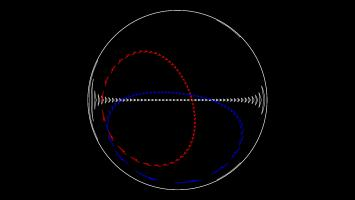### Craig retroazimuthal projection

A retroazimuthal projection makes a map on which the directions to the centre point from other points on the map are the same as the directions on the sphere. If you're thinking that this is the same as the azimuthal projection, then you're too used to doing geometry on flat surfaces: on a sphere, the sum of the angles in a triangle depends on the size of the triangle, so the directions from A to B and from B to A aren't as closely related as you would expect.
The Craig retroazimuthal projection was invented by James Ireland Craig in 1909. He used Mecca as his centre point to make a map that shows muslims across the world which direction they should face to pray.
The picture below shows what three spaceships travelling in a straight lines on a sphere looks like on this projection.
You can play Mathsteroids in Craig retroazimuthal mode here to explore the projection yourself. This is perhaps the hardest of all to play, as (a) two different parts of the sphere overlap on the map, and (b) the map is actually infinitely tall, so quite a bit of it is off the edge of the visible game area.

### Stereographic projection

The final projection I'd like to show you is the stereographic projection.
Imagine that a sphere is sitting on a 2D plane. Take a point on the sphere. Imagine a straight line through this point and the point at the top of the sphere. The point where this line meets the 2D plane is stereographic projection of the point on the sphere.
This projection (backwards) can be used to represent the every complex number as a point on a sphere: this is called the Riemann sphere.
To make Mathseteroids playable after this projection, I split the sphere into 2 hemisphere and projected each seperately to give two circles. You can play Mathsteroids in stereographic projection mode here. Three spaceships travelling in straight lines on this projection are shown below.
... and if you still don't like map projections, you can still enjoy playing Mathsteroids on an old fashioned torus. Or on a Klein bottle or the real projective plane. Don't forget to take a short break from playing to head over to The Aperiodical and vote (voting now closed).

### Similar postsVideo game surfaces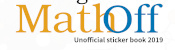Big Internet Math-Off stickers 2019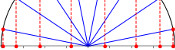Runge's PhenomenonBuilding MENACEs for other games

Comments in green were written by me. Comments in blue were not written by me.

Allowed HTML tags: <br> <a> <small> <b> <i> <s> <sup> <sub> <u> <spoiler> <ul> <ol> <li>
To prove you are not a spam bot, please type "y" then "-" then "a" then "x" then "i" then "s" in the box below (case sensitive):
2016-12-23
In many early arcade games, the size of the playable area was limited by the size of the screen. To make this area seem larger, or to make gameplay more interesting, many games used wraparound; allowing the player to leave one side of the screen and return on another. In Pac-Man, for example, the player could leave the left of the screen along the arrow shown and return on the right, or vice versa.
Pac-Man's apparent teleportation from one side of the screen to the other may seem like magic, but it is more easily explained by the shape of Pac-Man's world being a cylinder.
Rather than jumping or teleporting from one side to the other, Pac-Man simply travels round the cylinder.
Bubble Bobble was first released in 1986 and features two dragons, Bub and Bob, who are tasked with rescuing their girlfriends by trapping 100 levels worth of monsters inside bubbles. In these levels, the dragons and monsters may leave the bottom of the screen to return at the top. Just like in Pac-Man, Bub and Bob live on the surface of a cylinder, but this time it's horizontal not vertical.
A very large number of arcade games use left-right or top-bottom wrapping and have the same cylindrical shape as Pac-Man or Bubble Bobble. In Asteroids, both left-right and top-bottom wrapping are used.
The ships and asteroids in Asteroids live on the surface of a torus, or doughnut: a cylinder around to make its two ends meet up.
There is, however, a problem with the torus show here. In Asteroids, the ship will take amount of time to get from the left of the screen to the right however high or low on the screen it is. But the ship can get around the inside of the torus shown faster than it can around the outside, as the inside is shorter. This is because the screen of play is completely flat, while the inside and outside halves of the torus are curved.
It is impossible to make a flat torus in three-dimensional space, but it is possible to make one in four-dimensional space. Therefore, while Asteroids seems to be a simple two-dimensional game, it is actually taking place on a four-dimensional surface.
Wrapping doesn't only appear in arcade games. Many games in the excellent Final Fantasy series use wrapping on the world maps, as shown here on the Final Fantasy VIII map.
Just like in Asteroids, this wrapping means that Squall & co. carry out their adventure on the surface of a four-dimensional flat torus. The game designers, however, seem to not have realised this, as shown in this screenshot including a spherical (!) map.
Due to the curvature of a sphere, lines that start off parallel eventually meet. This makes it impossible to map nicely between a flat surface to a sphere (this is why so many different map projections exist), and heavily complicates the task of making a game with a truly spherical map. So I'll let the Final Fantasy VIII game designers off. Especially since the rest of the game is such incredible fun.
It is sad, however, that there are no games (at leat that I know of) that make use of the great variety of different wrapping rules available. By only slightly adjusting the wrapping rules used in the games in this post, it is possible to make games on a variety of other surfaces, such a Klein bottles or Möbius strips as shown below.
If you know of any games make use of these surfaces, let me know in the comments below!

### Similar posts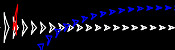MathsteroidsOptimal Pac-Man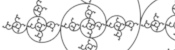Harriss and other spiralsMENACE in fiction

Comments in green were written by me. Comments in blue were not written by me.
2016-12-25
HyperRogue also has special modes which experiment with other geometries (spherical, and elliptic which is non-orientable). Hydra Slayer has Mobius strip and Klein bottle levels.
2016-12-24
HyperRogue is an example of a game that takes place on the hyperbolic plane. Rather than looping, the map is infinite.

See: http://zenorogue.blogspot.com.au/2012/03/hyperbolic-geometry-in-hyperbolic-rogue.html
2016-12-24
Hyperrogue may be the only game in existence with a hyperbolic surface topology: http://www.roguetemple.com/z/hyper/
2016-12-24
F-Zero GX had a track called Mobius Ring, that was... well, a M�bius ring.

F-Zero X had a more trivial track that was just the outward side of a regular ring, but it was rather weird too, because it meant that this was a looping track that had no turns.
2016-12-24
I don't know about video-games but there are puzzles by Jeff Weeks (http://www.geometrygames.org/) on torus.

Allowed HTML tags: <br> <a> <small> <b> <i> <s> <sup> <sub> <u> <spoiler> <ul> <ol> <li>
To prove you are not a spam bot, please type "tneitouq" backwards in the box below (case sensitive):
2016-03-31
Pythagoras's Theorem is perhaps the most famous theorem in maths. It is also very old, and for over 2500 years mathematicians have been explaining why it is true.
This has led to hundreds of different proofs of the theorem. Many of them were collected in the 1920s in The pythagorean proposition by Elisha Scott Loomis . Let's have a look at some of them.

### Using similar triangles

For our first proof, start with a right angled triangle, $$ABC$$, with sides of lengths $$a$$, $$b$$ and $$c$$.
Add a point $$D$$ on the hypotenuse such that the line $$AD$$ is perpendicular to $$BC$$. Name the lengths as shown in the second diagram.
$$ABC$$ and $$DBA$$ are similar triangles, so:
$$\frac{b}{x}=\frac{c}{b}$$ $$b^2=xc$$
$$ABC$$ and $$DAC$$ are similar triangles, so:
$$\frac{a}{c-x}=\frac{c}{a}$$ $$a^2=c^2-cx$$
$$a^2+b^2=c^2$$

This proof shows the theorem is true by using extra lines and points added to the triangle. Start with $$ABC$$ as before then add a point $$D$$ such that $$AD$$ and $$BC$$ are perpendicular and of equal length. Add points $$E$$ on $$AC$$ and $$F$$ on $$AB$$ (extended) such that $$DE$$ and $$AC$$ are perpendicular and $$DF$$ and $$AB$$ are perpendicular.
By similar triangles, it can be seen that $$DF=b$$ and $$DE=a$$.
As the two diagonals of $$BACD$$ are perpendicular, its area is $$\tfrac12c^2$$.
The area of $$BACD$$ is also equal to the sum of the areas of $$ABD$$ and $$ACD$$. The area of $$ABD$$ is $$\tfrac12b^2$$. The area of $$ACD$$ is $$\tfrac12a^2$$.
Therefore, $$\tfrac12a^2+\tfrac12b^2=\tfrac12c^2$$, which implies that $$a^2+b^2=c^2$$.

### Using a circle

This proof again uses extra stuff: this time using a circle. Draw a circle of radius $$c$$ centred at $$C$$. Extend $$AC$$ to $$G$$ and $$H$$ and extend $$AB$$ to $$I$$.
By the intersecting chord theorem, $$AH\times AG = AB\times AI$$. Using the facts that $$AI=AB$$ and $$CH$$ and $$CG$$ are radii, the following can be obtained from this:
$$(c-a)\times(c+a)=b\times b$$ $$c^2-a^2=b^2$$ $$a^2+b^2=c^2$$

### Rearrangement proofs

A popular method of proof is dissecting the smaller squares and rearranging the pieces to make the larger square. In both the following, the pieces are coloured to show which are the same:
Alternatively, the theorem could be proved by making copies of the triangle and moving them around. This proof was presented in The pythagorean proposition simply with the caption "LOOK":
This next proof uses the fact that two parallelograms with the same base and height have the same area: sliding the top side horizontally does not change the area. This allows us to move the smaller squares to fill the large square:
For this proof, start by labelling the sides of the triangle as vectors $$\alpha$$, $$\beta$$ and $$\gamma$$.
Clearly, $$\gamma = \alpha+\beta$$. Taking the dot product of each side with itself gives:
$$\gamma\cdot\gamma = \alpha\cdot\alpha+2\alpha\cdot\beta+\beta\cdot\beta$$
$$\alpha$$ and $$\beta$$ are perpendicular, so $$\alpha\cdot\beta=0$$; and dotting a vector with itself gives the size of the vector squared, so:
$$|\gamma|^2=|\alpha|^2+|\beta|^2$$
If you don't like any of these proofs, there are of course many, many more. Why don't you tweet me your favourite.

#### References

The pythagorean proposition by Elisha Scott Loomis. 1928. [link]

### Similar postsHarriss and other spiralsWorld Cup stickers 2018, pt. 3MathsteroidsVideo game surfaces

Comments in green were written by me. Comments in blue were not written by me.